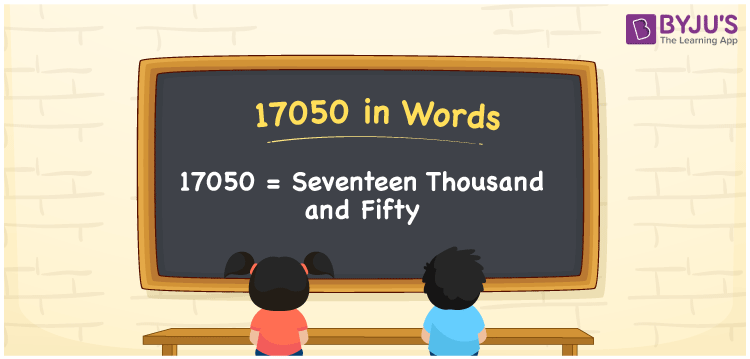# 17050 in Words

17050 in words is written as Seventeen thousand fifty. In both the International System of Numerals and the Indian System of Numerals, 17050 is written as Seventeen thousand fifty. The number 17050 is a Cardinal Number as it denotes some quantity. For example, “that mobile phone costs 17050 rupees”.

 17050 in Words Seventeen thousand fifty Seventeen thousand fifty in Number 17050

## 17050 in English Words

17050 in English words is read as “Seventeen thousand fifty”.## How to Write 17050 in Words?

To write 17050 in words, we shall use the place value chart. In the place value chart, put 1 in the ten thousands, 7 in the thousands, 0 in the hundreds, 5 in the tens and 0 in the ones. Let us make a place value chart to write the number 17050 in words.

 Ten Thousands Thousands Hundreds Tens Ones 1 7 0 5 0

Thus, we can write the expanded form as

1 × Ten Thousand + 7 × Thousand + 0 × Hundred + 5 × Ten + 0 × One

= 1 × 10000 + 7 × 1000 + 0 × 100 + 5 × 10 + 0 × 1

= 10000 + 7000 + 0 +50 + 0

= 17050

= Seventeen thousand fifty.

17050 is a natural number, the successor of 17049 and the predecessor of 17051.

17050 in words – Seventeen thousand fifty

• Is 17050 an odd number? – No
• Is 17050 an even number? – Yes
• Is 17050 a perfect square number? – No
• Is 17050 a perfect cube number? – No
• Is 17050 a prime number? – No
• Is 17050 a composite number? – Yes

## Frequently Asked Questions on 17050 in Words

Q1

### How to write 17050 in words?

17050 in words is written as Seventeen thousand fifty.
Q2

### How to write 17050 in the International and Indian System of Numerals?

In both, the system of numerals, 17050 in words, is written as Seventeen thousand fifty.
Q3

### How to write 17050 in a place value chart?

In the place value chart, write 1 in the ten thousands, 7 in the thousands, 0 in the hundreds, 5 in the tens and 0 in the ones, respectively.